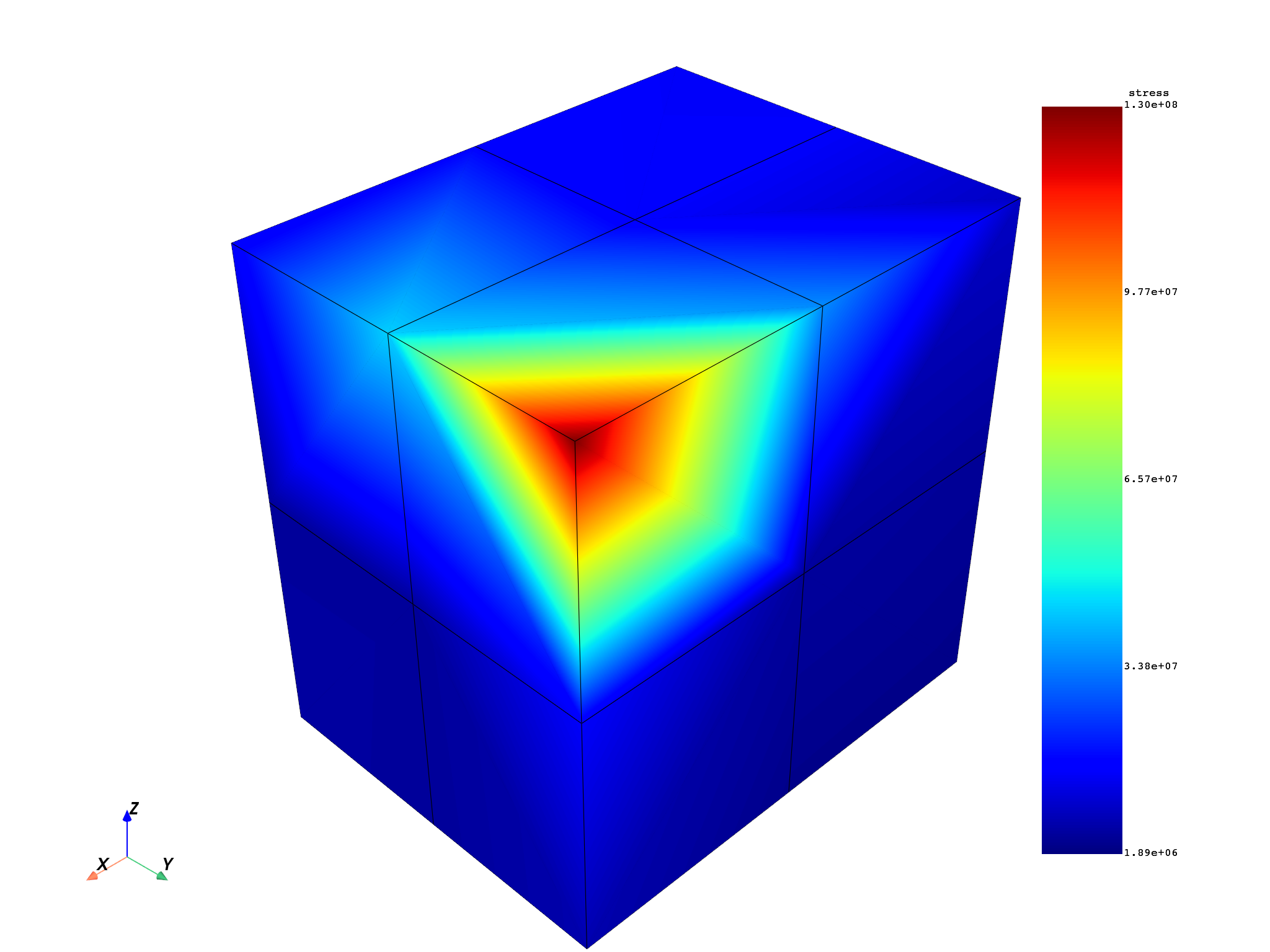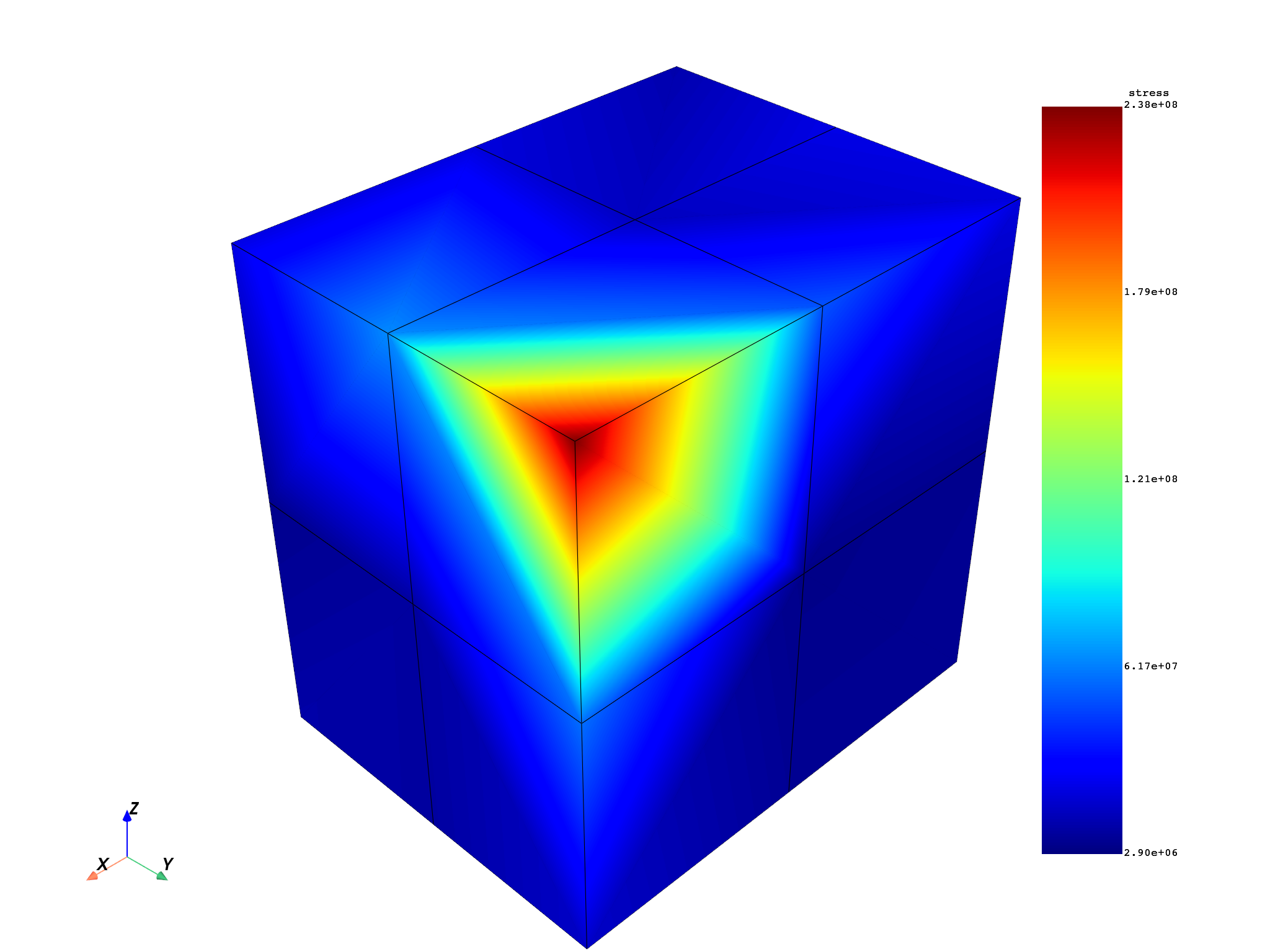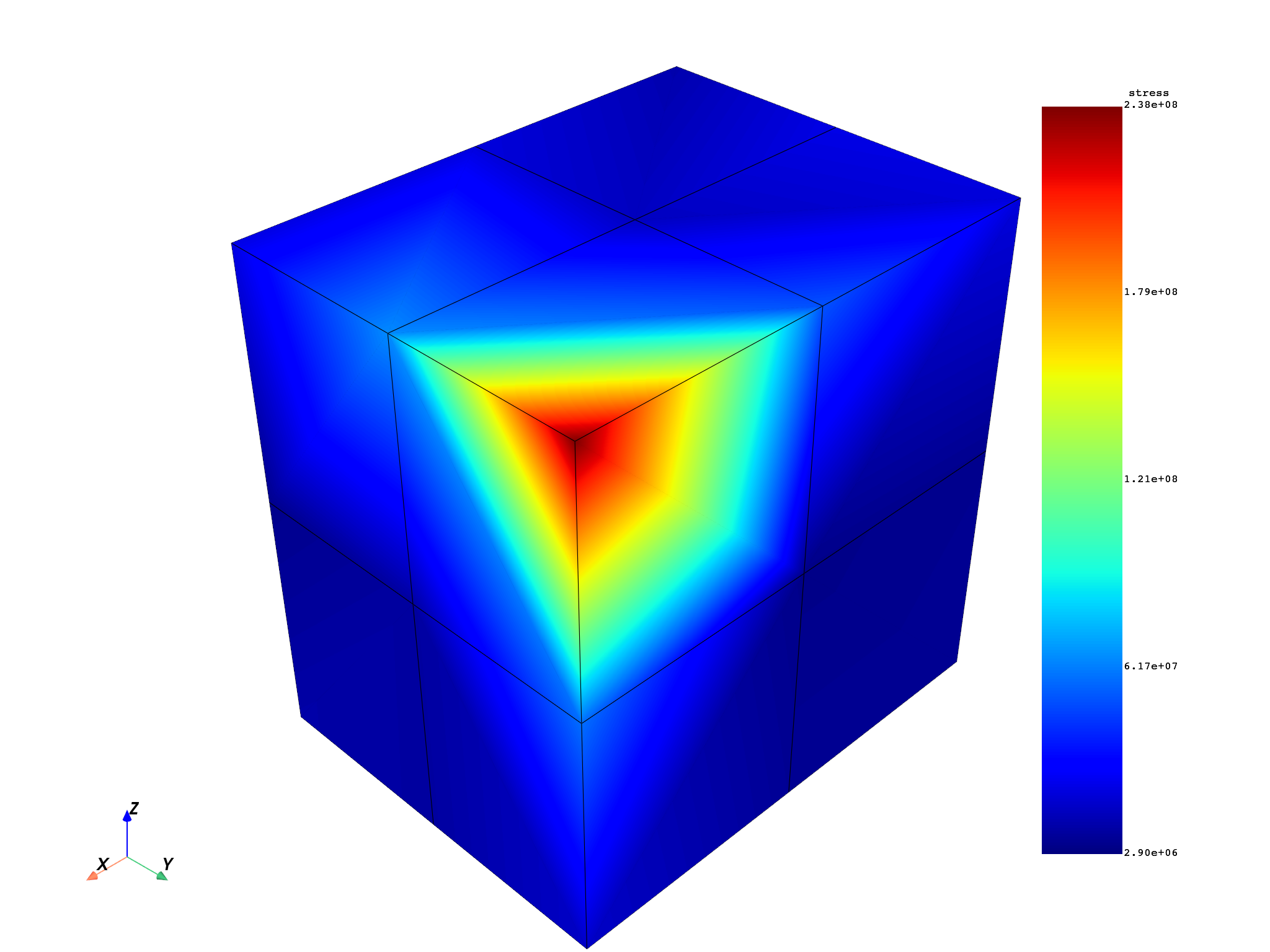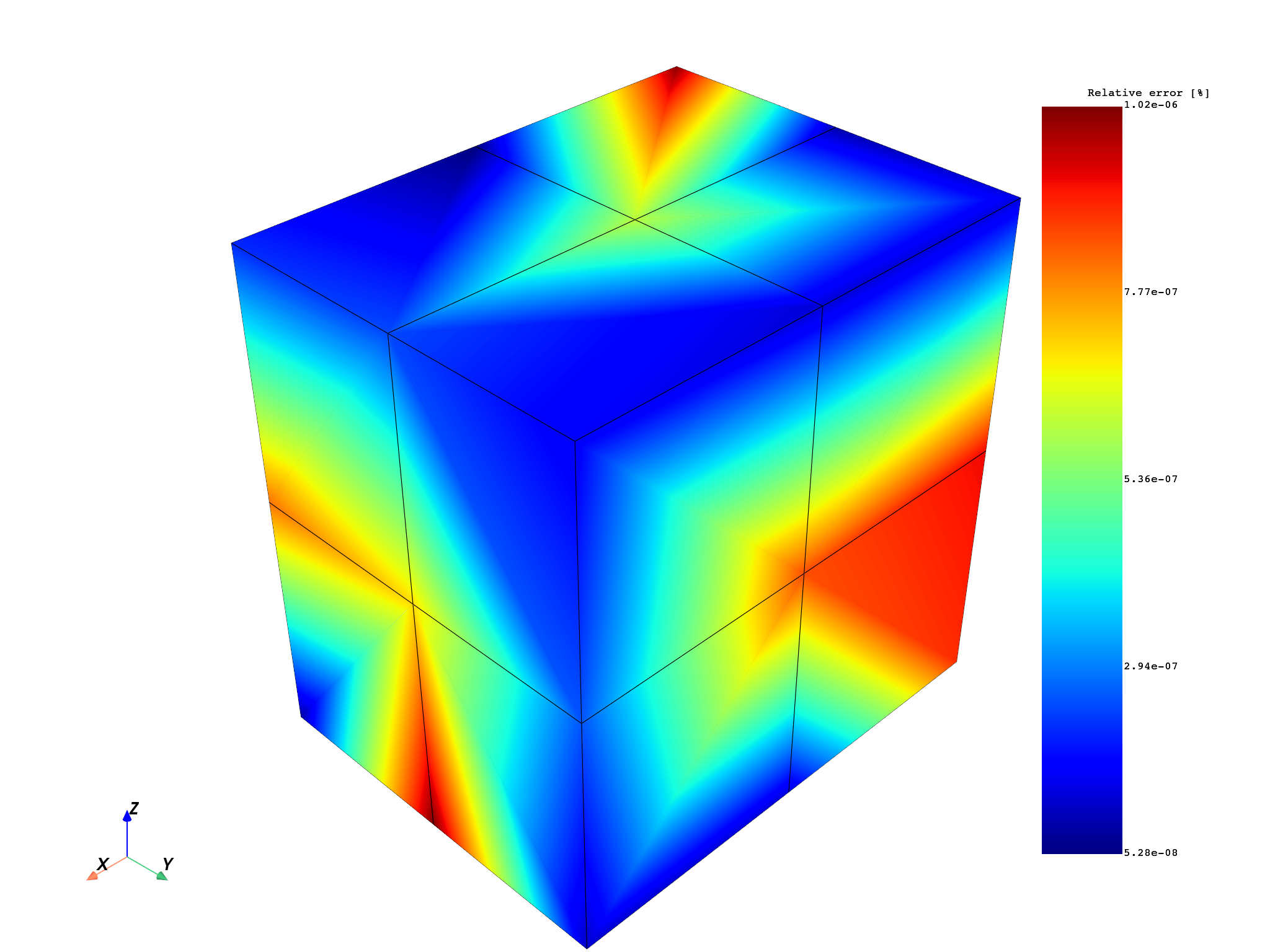# Extrapolation method for stress result of a 3D element#

This example shows how to compute the stress nodal components from Gaussian points (integration points) for a 3D element using extrapolation.

Extrapolate results available at Gaussian or quadrature points to nodal points for a field or fields container. The available elements are:

• Linear hexagonal

• Linear tetrahedral

Here are the steps for extrapolation:

1. Get the data source’s solution from the integration points. (This result file was generated with the Ansys Mechanical APDL (MAPDL) option `ERESX, NO`).

2. Use the extrapolation operator to compute the nodal stress.

3. Get the result for nodal stress from the data source. The analysis was computed by MAPDL.

4. Compare the result for nodal stress from the data source and the nodal stress computed by the extrapolation method.

```from ansys.dpf import core as dpf
from ansys.dpf.core import examples
```

Get the data source’s analysis of integration points and analysis reference

```datafile = examples.download_extrapolation_3d_result()

# Get integration points (Gaussian points)
data_integration_points = datafile["file_integrated"]
data_sources_integration_points = dpf.DataSources(data_integration_points)

# Get the reference
dataSourceref = datafile["file_ref"]
data_sources_ref = dpf.DataSources(dataSourceref)

# Get the mesh
model = dpf.Model(data_integration_points)

# Operator instantiation scoping
op_scoping = dpf.operators.scoping.split_on_property_type()  # operator instantiation
op_scoping.inputs.mesh.connect(mesh)
op_scoping.inputs.requested_location.connect("Elemental")
mesh_scoping = op_scoping.outputs.mesh_scoping()
```

# Extrapolate from integration points for stress result#

This example uses the `gauss_to_node_fc` operator to compute the nodal component stress result from the stress result of integration points.

```# Create stress operator to get stress result of integration points
stressop = dpf.operators.result.stress()
stressop.inputs.data_sources.connect(data_sources_integration_points)
stress = stressop.outputs.fields_container()
```

## Nodal stress result of integration points#

The MAPLD command `ERESX,NO` is used to copy directly the Gaussian (integration) points results to the nodes, instead of the results at nodes or elements (which are interpolation of results at a few gauss points). The following plot shows the nodal values, which are the averaged values of stresses at each node. The value shown at the node is the average of the stresses from the Gaussian points of each element that it belongs to.

```# Plot
stress_nodal_op = dpf.operators.averaging.elemental_nodal_to_nodal_fc()
stress_nodal_op.inputs.fields_container.connect(stress)
mesh.plot(stress_nodal_op.outputs.fields_container())
```Create operator `gauss_to_node_fc` and compute nodal component stress by applying the extrapolation method.

```ex_stress = dpf.operators.averaging.gauss_to_node_fc()
# connect mesh
ex_stress.inputs.mesh.connect(mesh)
# connect fields container stress
ex_stress.inputs.fields_container.connect(stress)
# get output
fex = ex_stress.outputs.fields_container()
```

# Stress result of reference Ansys Workbench#

```# Stress from file dataSourceref
stressop_ref = dpf.operators.result.stress()
stressop_ref.inputs.data_sources.connect(data_sources_ref)
stressop_ref.inputs.mesh_scoping.connect(mesh_scoping)
stress_ref = stressop_ref.outputs.fields_container()
```

# Plot#

Show plots of the extrapolation’s stress result and the reference’s stress result

```# extrapolation
fex_nodal_op = dpf.operators.averaging.elemental_nodal_to_nodal_fc()
fex_nodal_op.inputs.fields_container.connect(fex)
fex_nodal_fc = fex_nodal_op.eval()
mesh.plot(fex_nodal_fc)

# reference
stress_ref_nodal_op = dpf.operators.averaging.elemental_nodal_to_nodal_fc()
stress_ref_nodal_op.inputs.fields_container.connect(stress_ref)
stress_ref_nodal_fc = stress_ref_nodal_op.eval()
mesh.plot(stress_ref_nodal_fc)
```
••# Compare stress results#

Compare the stress result computed by extrapolation and the reference’s result. Check if the two fields container are identical using the `identical_fc` operator. The relative tolerance is set to 1.1e-6. The smallest value that is considered during the comparison step: all the `abs(values)` in field less than 1e-2 is considered as null.

```# operator AreFieldsIdentical_fc
op = dpf.operators.logic.identical_fc()
op.inputs.fields_containerA.connect(fex_nodal_op)
op.inputs.fields_containerB.connect(stress_ref_nodal_op)
op.inputs.tolerance.connect(1.1e-6)
op.inputs.small_value.connect(0.01)
op.outputs.boolean()
```
```True
```

Compute absolute and relative errors

```abs_error_sqr = dpf.operators.math.sqr_fc()
abs_error = dpf.operators.math.sqrt_fc()
error = stress_ref_nodal_op - fex_nodal_op
abs_error_sqr.inputs.fields_container.connect(error)
abs_error.inputs.fields_container.connect(abs_error_sqr)

divide = dpf.operators.math.component_wise_divide()
divide.inputs.fieldA.connect(stress_ref_nodal_op - fex_nodal_op)
divide.inputs.fieldB.connect(stress_ref_nodal_op)
rel_error = dpf.operators.math.scale()
rel_error.inputs.field.connect(divide)
rel_error.inputs.ponderation.connect(1.0)
```

Plot absolute and relative errors. The absolute value is the order of 10, which is very small when compared to the magnitude of 1e8 of the displacements. This is reflected in the relative error plot, where the errors are found to be below 1.02e-6%. The result of these plots can be used to set the tolerances for the `identical_fc` operator.

```mesh.plot(abs_error.eval(), scalar_bar_args={"title": "Absolute error [mm]"})
mesh.plot(rel_error.eval(), scalar_bar_args={"title": "Relative error [%]"})
```
••Total running time of the script: ( 0 minutes 10.858 seconds)

Gallery generated by Sphinx-Gallery